# How to Load a Massive File as small chunks in Pandas?

• Last Updated : 01 Oct, 2020

Pandas in flexible and easy to use open-source data analysis tool build on top of python which makes importing and visualizing data of different formats like .csv, .tsv, .txt and even .db files.

For the below examples we will be considering only .csv file but the process is similar for other file types. The method used to read CSV files is read_csv()

Parameters:

filepath_or_bufferstr : Any valid string path is acceptable. The string could be a URL. Valid URL schemes include http, ftp, s3, gs, and file. For file URLs, a host is expected. A local file could be: file://localhost/path/to/table.csv.

iteratorbool : default False Return TextFileReader object for iteration or getting chunks with get_chunk().

chunksize : int, optional Return TextFileReader object for iteration. See the IO Tools docs for more information on iterator and chunksize.

The read_csv() method has many parameters but the one we are interested is chunksize. Technically the number of rows read at a time in a file by pandas is referred to as chunksize. Suppose If the chunksize is 100 then pandas will load the first 100 rows. The object returned is not a data frame but a TextFileReader which needs to be iterated to get the data.

In the below program we are going to use the toxicity classification dataset which has more than 10000 rows. This is not much but will suffice for our example.

## Python3

 `import` `pandas as pd``from` `pprint ``import` `pprint`` ` `df ``=` `pf.read_csv(``'train/train.csv'``)`` ` `df.columns`

Output: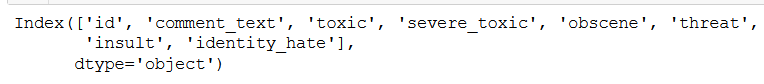First Lets load the dataset and check the different number of columns. This dataset has 8 columns.

Let’s get more insights about the type of data and number of rows in the dataset.

## Python3

 `df.info()`

Output: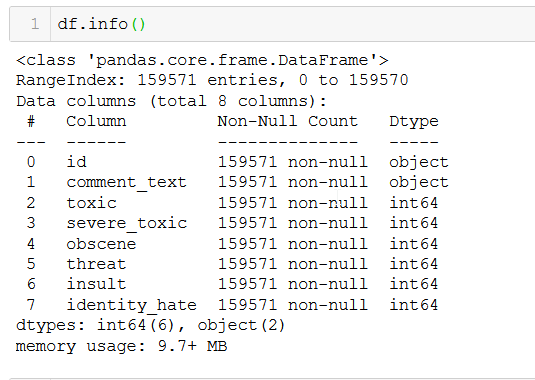We have a total of 159571 non-null rows.

## Python3

 `df ``=` `pd.read_csv(``"train/train.csv"``, chunksize``=``10000``)``print``.``print``(df)`

Output: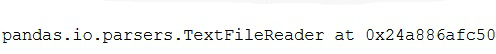Here we are creating a chunk of size 10000 by passing the chunksize parameter. The object returned is not a data frame but an iterator, to get the data will need to iterate through this object.

## Python3

 `for` `data ``in` `df:``    ``pprint(data.shape)`

Output: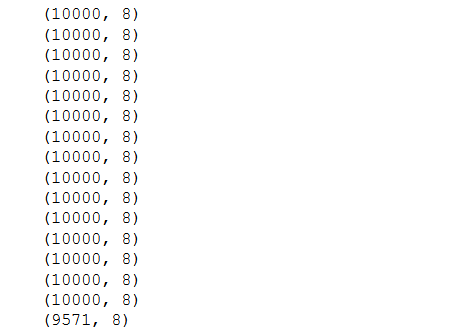Now, calculating the number of chunks-

## Python3

 `df ``=` `pd.read_csv(``"train/train.csv"``, chunksize``=``10``)`` ` `for` `data ``in` `df:``    ``pprint(data)``    ``break`

Output: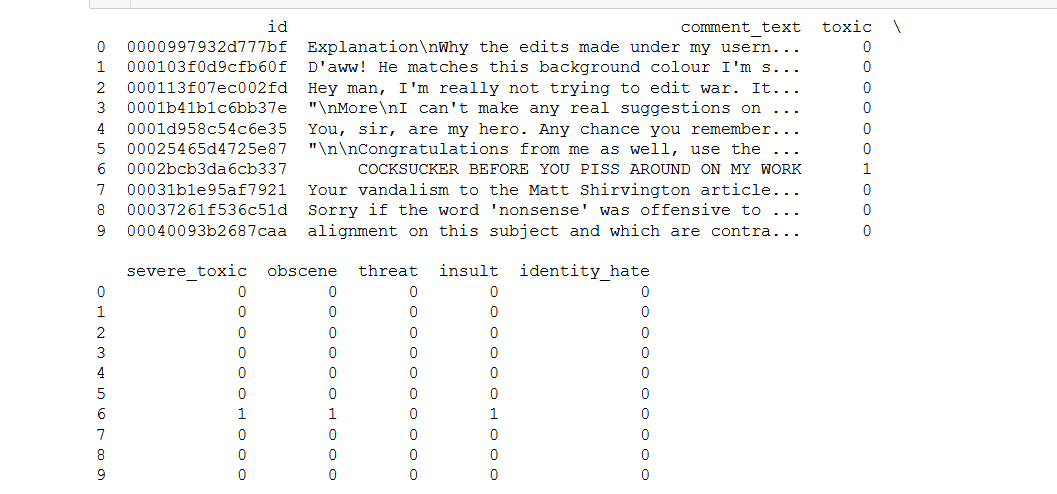In the above example, each element/chunk returned has a size of 10000.  Remember we had 159571. Hence, the number of chunks is 159571/10000 ~ 15 chunks, and the remaining 9571 examples form the 16th chunk.

The number of columns for each chunk is 8. Hence, chunking doesn’t affect the columns. Now that we understand how to use chunksize and obtain the data lets have a last visualization of the data, for visibility purposes, the chunk size is assigned to 10.

My Personal Notes arrow_drop_up